Latest Banking jobs   »

# Quantitative Aptitude Quiz For SIDBI Grade A Prelims 2023- 20th January

Q1. Veer borrows Rs. 6000 at 30% compound rate of interest. At the end of each year he pays back Rs. 1200. How much amount should he pay at the end of the third year to clear all his dues?
(a) Rs. 9594
(b) Rs. 9584
(c) Rs. 9850
(d) Rs. 8574
(e) Rs. 8926

Q2. The ratio of age of Satish and his son is 7 : 2. If the difference of their ages 7 year ago is 25. Then find the sum of ages of Satish and his son 12 year hence ?
(a) 79
(b) 72
(c) 69
(d) 59
(e) 63

Q3. There are 150 student in a class, all the student appeared in a particular test. 33⅓% of student got a score of 70 as an average, ⅖th of the remaining scored 25 as an average and remaining scored 55 as an average marks. Then find the average marks of whole class ?
(a) 54
(b) 52
(c) 62
(d) 58
(e) 48

Q4. Arun and Veer together can complete a work in 15 days. Veer and Abhi together complete the same work in 12 days. If Arun started to work and worked for first 8 days alone and next 12 days Veer worked alone and the remaining work is completed by Abhi in 6 days. Then in how many days Veer alone will compete the whole work ?
(a) 70 days
(b) 40 days
(c) 60 days
(d) 64 days
(e) 55 days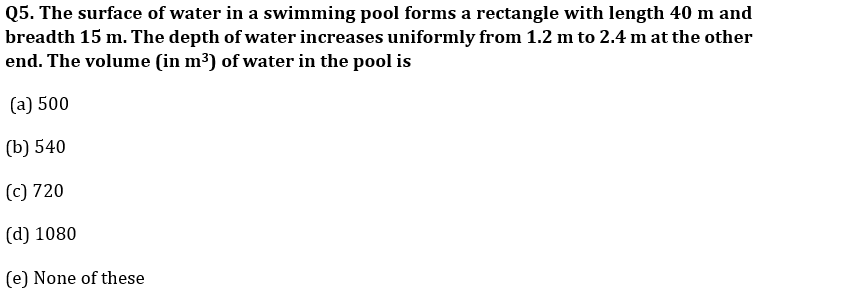Q6. If the cost of pins reduces by Rs. 4 per dozen, 12 more pins can be purchased for Rs. 48. The cost of pins per dozen after r

eduction is :
(a) Rs. 8
(b) Rs. 12
(c) Rs. 16
(d) Rs. 20
(e) Rs. 14

Q7. A box contains 30 eggs out of which 6 are rotten. Two eggs are chosen at random. What is the probability that only one of the chosen eggs is rotten ?
(a) 53/145
(b) 63/145
(c)51/145
(d) 57/145
(e) 48/145

Q8. Monthly incomes of A and B are in the ratio of 4 : 3 and their expenses bear the ratio 3 : 2. Each of them saves Rs. 6000 at the end of the month, then the monthly income of A is
(a) Rs. 12000
(b) Rs. 24000
(c) Rs. 30000
(d) Rs. 60000
(e) Rs. 28000

Q9. A and B started a business together with capital of Rs. 8000 and Rs. 10,000 respectively. A left the business after 5 months while B continued. A gets 20% of total profit for managing business and remaining is distributed in ratio of their profit sharing. If after a year, A gets total profit of Rs.2500 then, find the total profit earned by ‘A’ and ‘B’ together.
(a) 10,000
(b) 8,500
(c) 7,500
(d) 6,250
(e) 5,750

Q10. A box contains ‘x’ blue balls, 5 red and 5 black balls. If probability of choosing 2 blue balls from the bag is 0.125, then find the total number of balls in the box?
(a) 25
(b) 15
(c) 16
(d) 18
(e) 21

Q11. A society organised an event every Saturday of week and after the event sweets are distributed among member of society. Each male got two sweets and each female got three sweets. If three males leave society and 216 sweets are distributed, then ratio between total sweets got by male to female of society is 5 : 7. Find difference between number of male and female in society?
(a) 1
(b) 3
(c) 6
(d) 7
(e) 11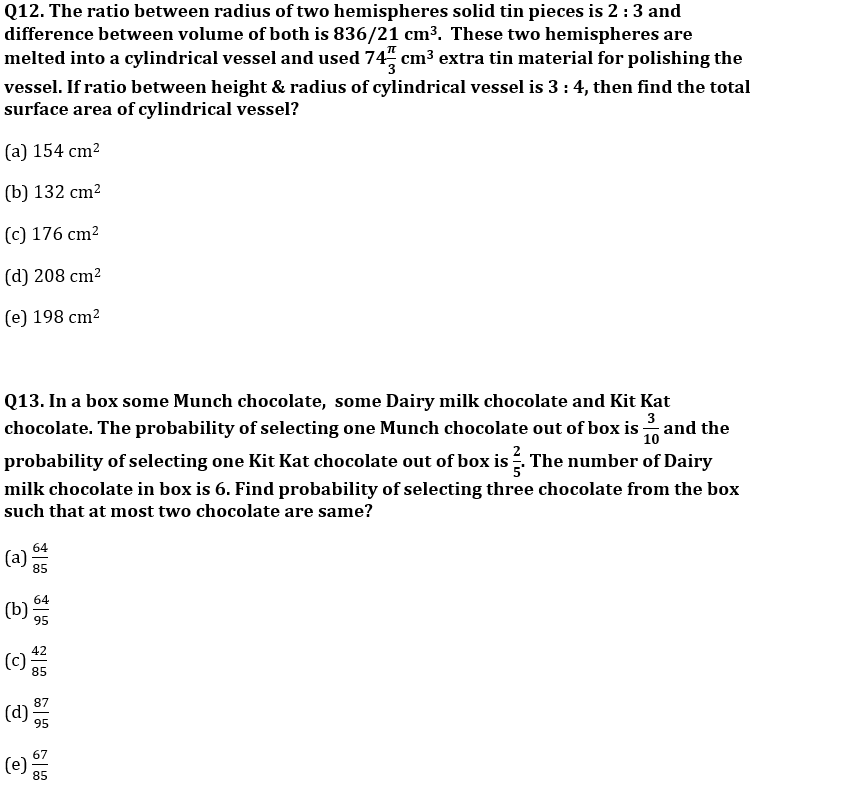Q14.Two pipes P and Q can fill tank A in 28 minutes and 56 minutes respectively and empty pipe M can empty the tank in 42 minutes. Tank A have the capacity of 168 liters. If all three pipes opened in tank B for (x – 24) minutes together they filled 90 liter of the tank which is 25% of the quantity of tank B. Find in x minutes what portion of tank B filled, if all pipe P and Q and M opened alternatively in each minute starting with P, followed by Q and M respectively?
(a) 5/36
(b) 7/36
(c) 9/38
(d) 7/38
(e) 7/39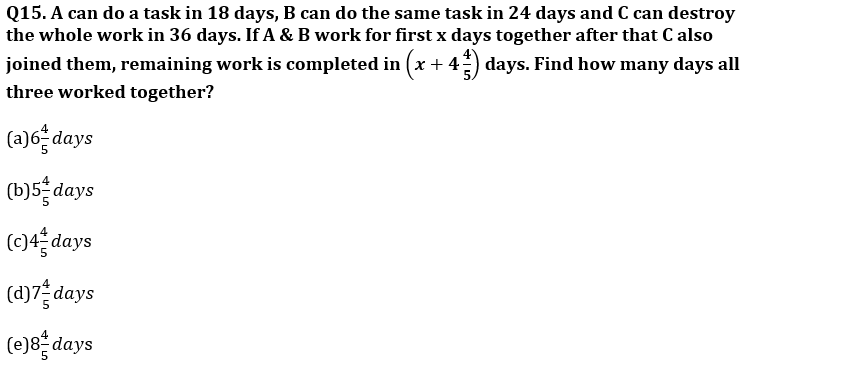Solutions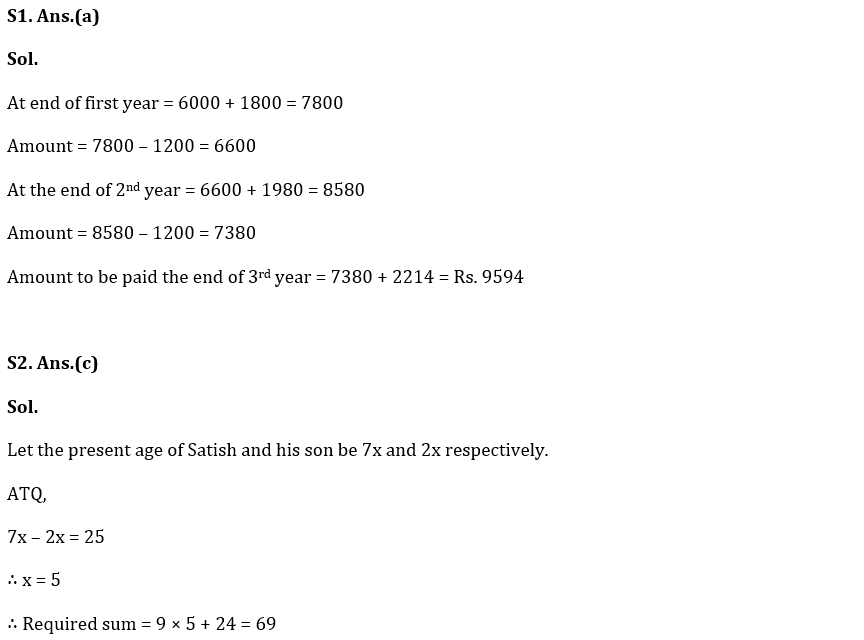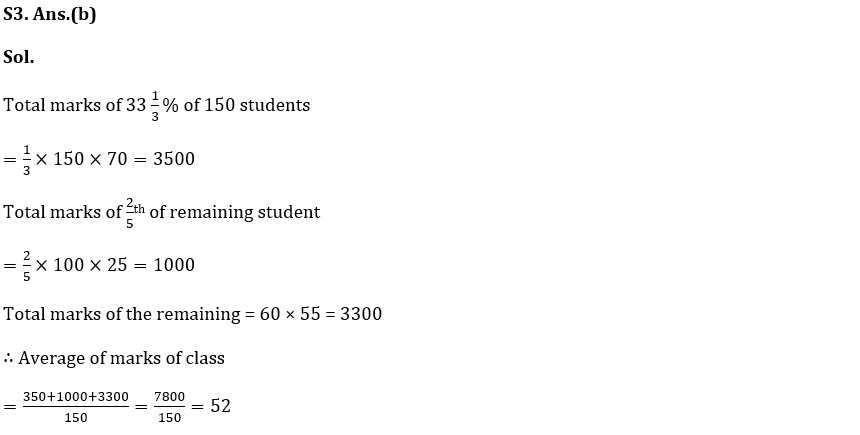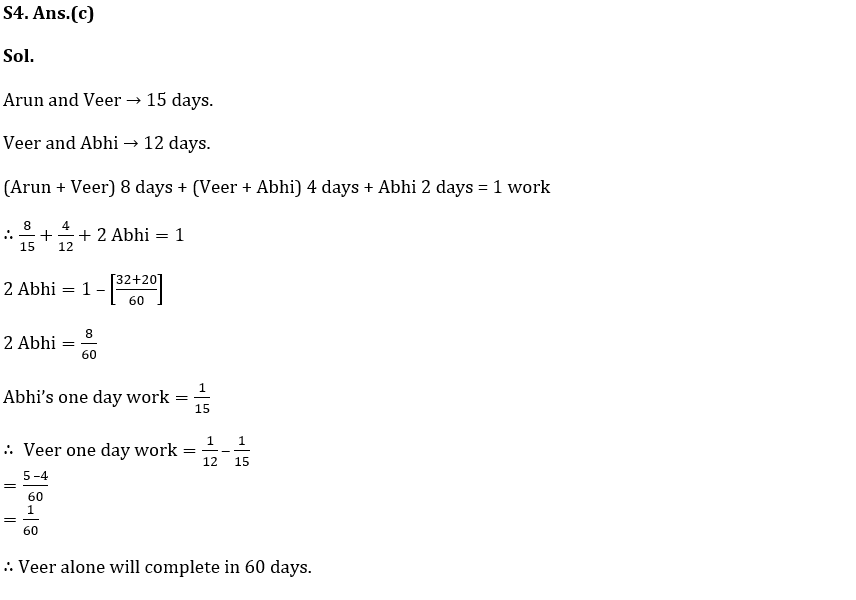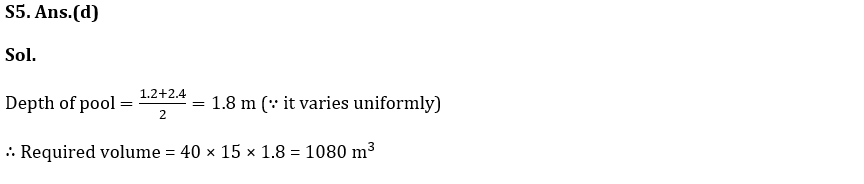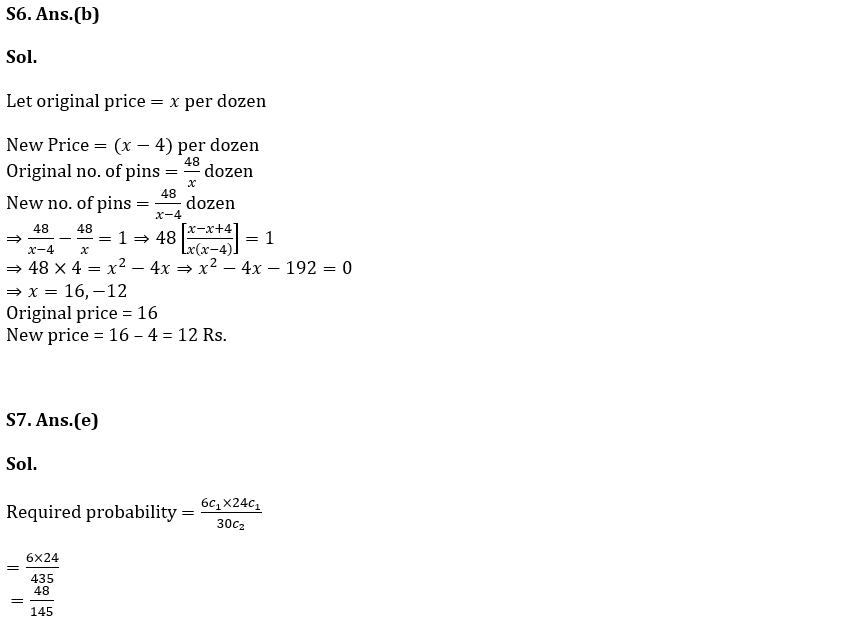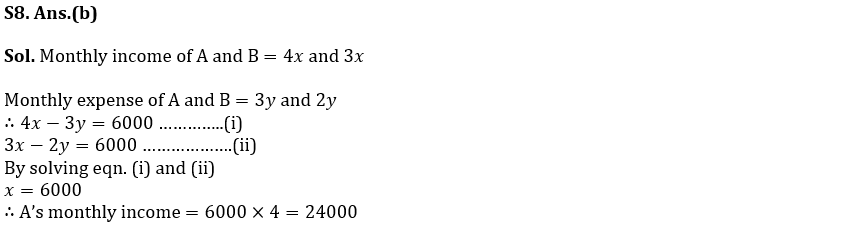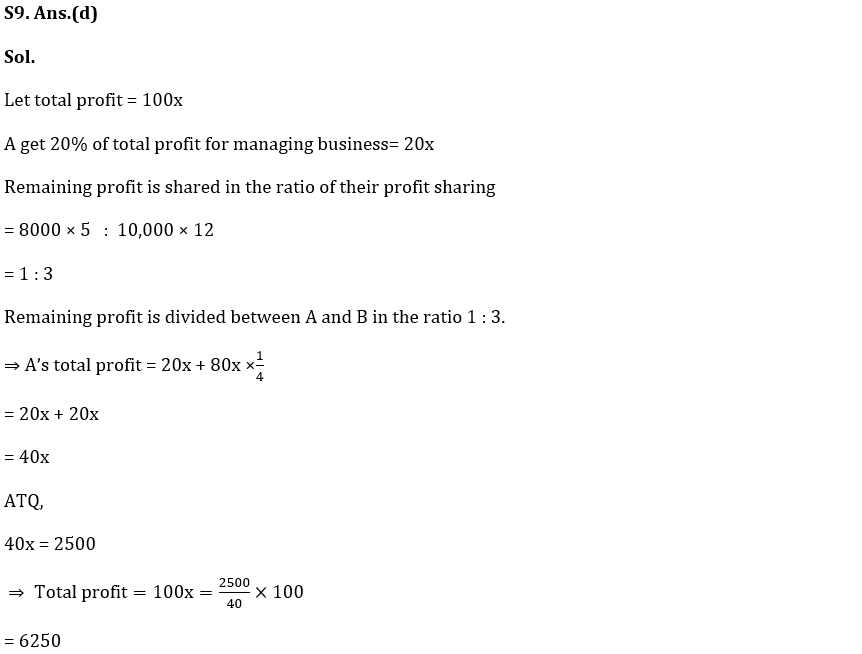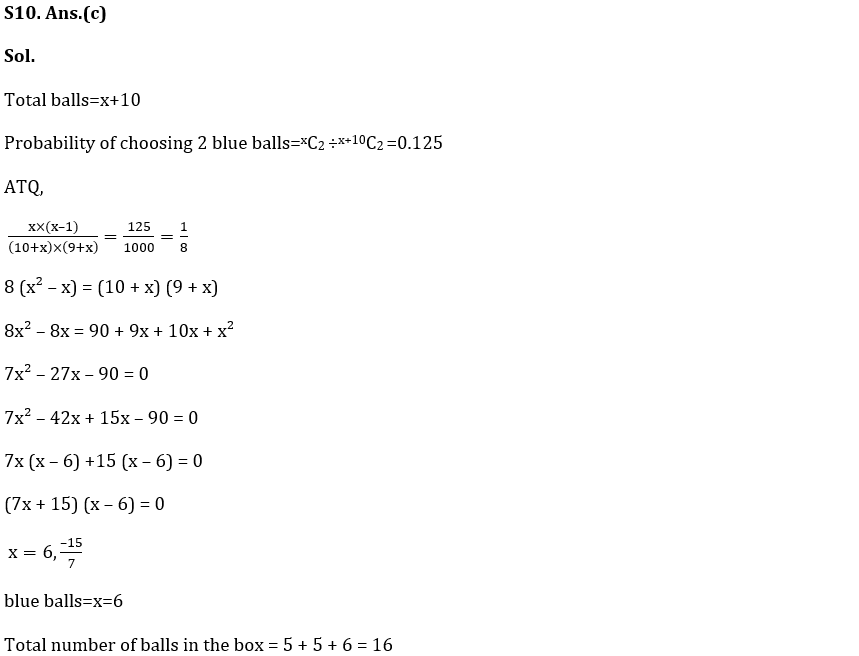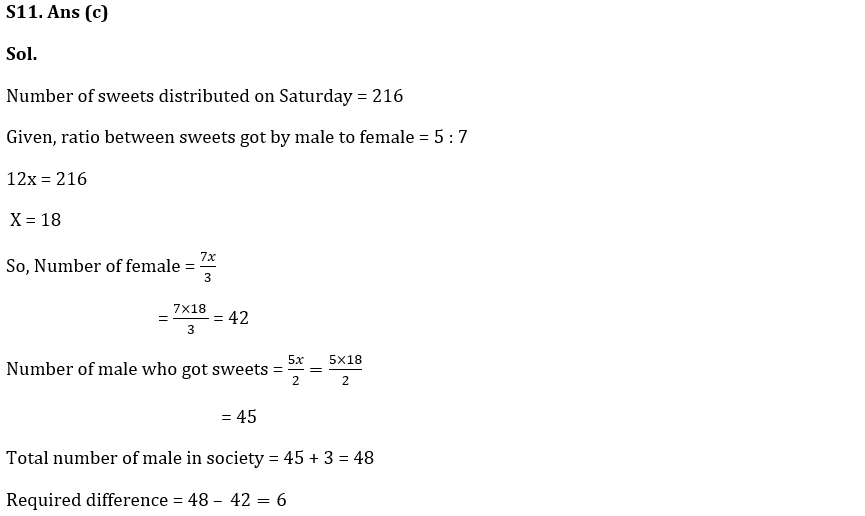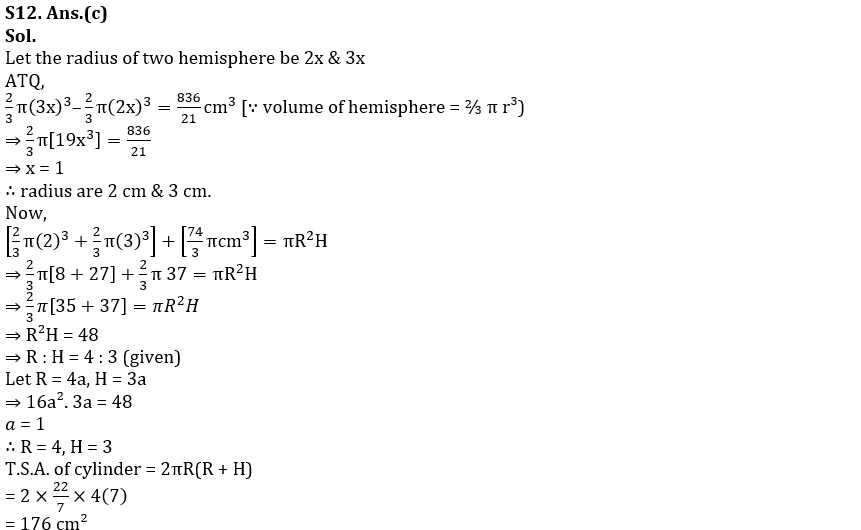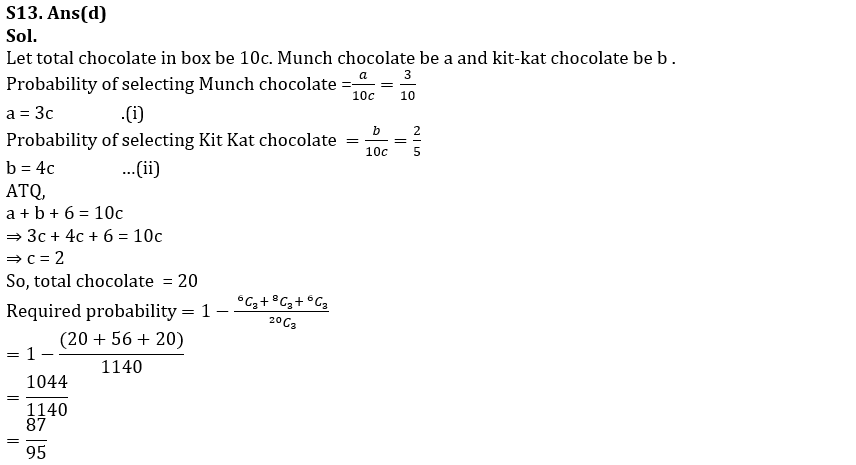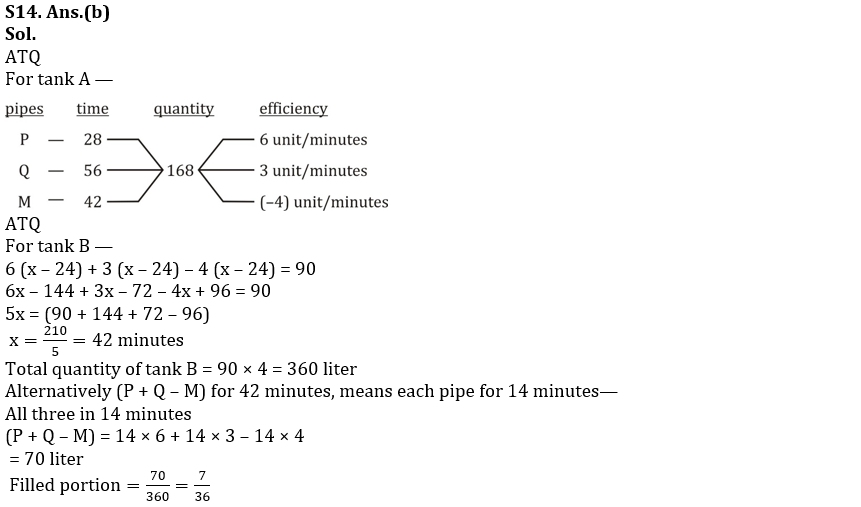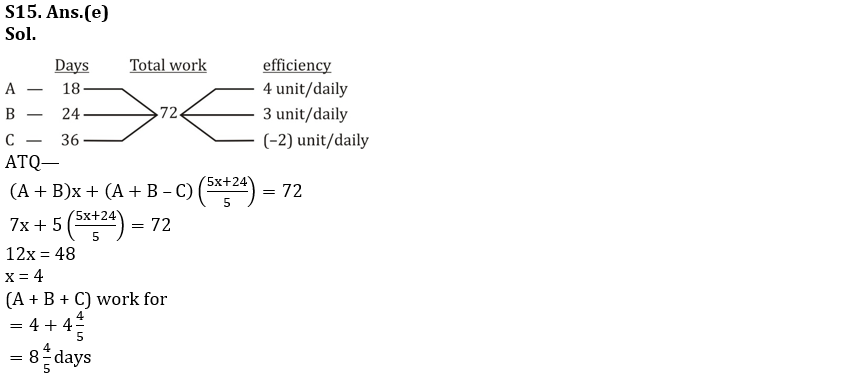## FAQs

### When will the SIDBI Grade A Exam 2023 be held?

The SIDBI Grade A Exam 2023 will be held on 28th January 2023.

#### Congratulations!Union Budget 2023-24: Free PDF